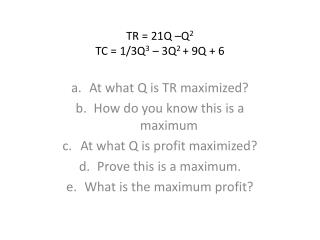DownloadDownload PresentationTR = 21Q –Q 2 TC = 1/3Q 3 – 3Q 2 + 9Q + 6

# TR = 21Q –Q 2 TC = 1/3Q 3 – 3Q 2 + 9Q + 6

Télécharger la présentation## TR = 21Q –Q 2 TC = 1/3Q 3 – 3Q 2 + 9Q + 6

- - - - - - - - - - - - - - - - - - - - - - - - - - - E N D - - - - - - - - - - - - - - - - - - - - - - - - - - -
##### Presentation Transcript

1. TR = 21Q –Q2TC = 1/3Q3 – 3Q2 + 9Q + 6 At what Q is TR maximized? How do you know this is a maximum At what Q is profit maximized? Prove this is a maximum. What is the maximum profit?

2. TR = 50Q – Q2 TC = 100 – 4Q + 2Q2 • A) At what Q is profit maximized? • B) Prove this is a maximum. • C) What is the total profit?

3. Demand curve is Q = 220 – PTC = 1000 + 80Q – 3Q2 + 1/3Q3 • A) Write the equation for Total Revenue. • B) Determine the output level and price that will maximize profit/minimize loss. • C) What is the profit/loss at this point?

4. Short run Total Cost STC = 4850 + 40Q – 1.5Q2 + 0.04Q3 • A) What is the dollar value of average fixed cost at an output of 25 units? • B) At what level will marginal cost be minimized? • C) Prove this is a minimum. • D) At what level will AVC be minimized? • E) Prove this is a minimum.

5. Demand function Q = 350 – 0.25PSTC = 20000 + 200Q – 9Q2 + 1/3Q3 • Determine the profit maximizing quantity sold and the price. • Prove this is a maximum. • What is the level of profit?

6. Demand Q = 75 – 0.5PSTC = 500 + 30Q – 3Q2+ 1/3Q3 • Find the MR equation for the firm. • Solve for the profit maximizing quantity and price.